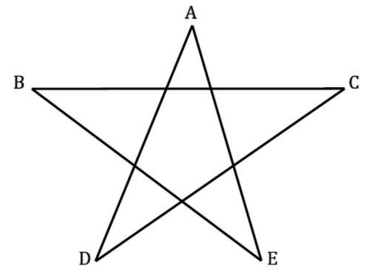# Twinkle Twinkle Angled Star

Geometry Level 2For a five pointed star as shown, compute the value of $\large \angle A + \angle B + \angle C + \angle D + \angle E .$

×